# Verbal Reasoning - Character Puzzles

### Exercise :: Character Puzzles - Character Puzzles 1

16.

Which one will replace the question mark ?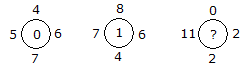A. 0 B. 2 C. 11 D. 12

Explanation:

(6 + 5) - (7 + 4) = 0

and (7 + 6) - (8 + 4) = 1

Therefore (11 + 2) - (2 + 0) = 11.

17.

Which one will replace the question mark ?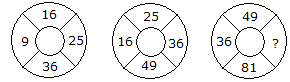A. 64 B. 144 C. 169 D. 25

Explanation:

The numbers are squared in ascending order

In first circle,

(3)2 = 9

(4)2 = 16

(5)2 = 25

(6)2 = 36.

In second circle,

(4)2 = 16

(5)2 = 25

(6)2 = 36

(7)2 = 49.

In third circle,

(6)2 = 36

(7)2 = 49

(8)2 = 64

(9)2 = 81.

18.

Which one will replace the question mark ?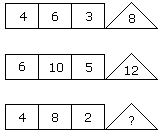A. 8 B. 12 C. 16 D. 20

Explanation:

(4 x 6) % 3 = 8

(6 x 10) % 5 = 12

(4 x 8) % 2 = 16.

19.

Which one will replace the question mark ?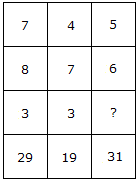A. 3 B. 5 C. 4 D. 6

Explanation:

(7 x 3) + 8 = 29

(4 x 3) + 7 = 19

(5 x ?) + 6 = 31

? = 5.

20.

Which one will replace the question mark ?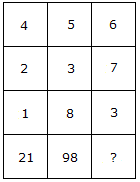A. 94 B. 76 C. 16 D. 73

Explanation:

(4)2 + (2)2 + (1)2 = 21

and (5)2 + (3)2 + (8)2 = 98

Therefore (6)2 + (7)2 + (3)2 = 94.